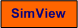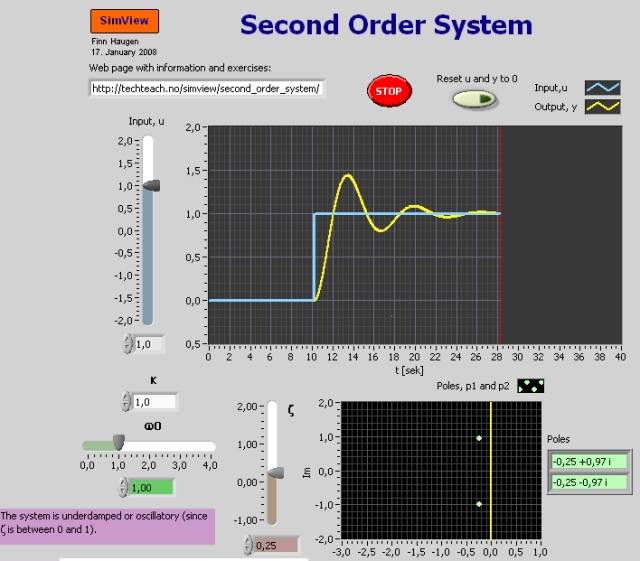# Second Order System

Snapshot of the front panel of the simulator:## Description of the system to be simulated

In this lab the step response of a general or standard second order system is simulated (that is, the time response on the output of the system is calculated numerically).

The mathematical model of the second order system which is simulated in this lab, is the differential equation

d2y/dt2 + 2zw0dy/dt + w02y =  w02Ku    (Eq. 1)

where

• K is the gain
• z is the relative damping factor
• w0 is the natural resonance frequency

An alternative way of representing this model is by Laplace transforming the differential equation, and taking the ratio of output to input (in the Laplace domain), to get the transfer function from input u to output y:

h(s) = y(s)/u(s) =  Kw02/(s2 + 2zw0s + w02)    (Eq. 2)

## Aim

To develop a qualitative understanding of how the following (standard) parameters influate the step respons of a second order system: Gain K, relative damping factor z, natural (or undamped) resonance frequency w0, and the step input height U.

## Motivation

Second order systems - as first order systems - constitute an important class of dynamic systems: There are many physical systems which behave (approximately) as second first order systems, e.g. stirred liquid tanks with thermal capacities in the liquid and in the heating element, DC-motors with armature dynamics and electro-mechanical dynamics, feedback control systems (e.g. process control systems and servomechanisms), sensors, and second order lowpass filters.

The equality sign "=" in the following text can be regarded as "approximately equal to" (so that you do not have to enter exact numeric values).

In several taskes below you are to give a value for the response-time, Tr. It is a convenient parameter to characterize how quick is a dynamic system with respect to it's step response. Tr is defined as the time it takes the step response to reach 63% of it's stationary (final) value. (For first order systems the response-time is equal to the time-constant.) It can be shown that for second order systems Tr is approximately equal to 1.5/w0 (it actually varies somewhat with z).

1. Impact of K: Set U=1, z=0.2, w0=2. Adjust K.
1. What is the impact of K on the stationary response?
2. What is the impact of T on the response-time, Tr?

2. Importance of z: Set U=1, K=2, w0=2. Adjust z.
1. What is the impact of z on the overshoot?
2. What is the impact of z on the response-time Tr?
3. What is the impact of z on the stationary response?
4. What is the impact of z on the stability of the system?

3. Importance of w0: Set U=1, K=2, z=0.2. Adjust w0.
1. What is the impact of w0 on the response-time Tr?
2. What is the impact of w0 on the stationary response?
3. What is the impact of w0 on the overshoot?
4. Adjust U up and down continuously (like a sinusoidal variation) for a small and for a large value of w0. How does w0 influate the "speed" of the system?

4. Importance of step height U: Set K=2, z=0.2, w0=2. Adjust U. What is the impact of U on the stationary response?

[SimView] [TechTeach]

Updated 27 August 2017. Developed by Finn Haugen. E-mail: finn@techteach.no.